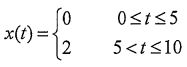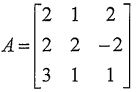106 年 - 106 國立中山大學_碩士班招生考試_資工系(乙組)：工程數學#105789

【非選題】
1.
1.If A is an n x n matrix, then A is called idempotent if A2 = A. Let A and B be n x n idempotent matrices.

【題組】 1.1 Show that AB is idempotent if AB = BA.

【非選題】
2.【題組】

1.2 Show that if A is idempotent, thenis idempotent.

【非選題】

【非選題】
4.【題組】1.4Find all values of k for which kA is also idempotent.

【非選題】
5.

2. A periodic signal x(t) with a period T0= 10, 0 ≤t ≤ 10 by the equation【題組】2.1 Sketch the periodic function x(t) over the time interval -10 s t ≤ 20.

【非選題】
6.【題組】2.2 Determine the DC coefficient of the Fourier series, ao.

【非選題】
7.【題組】2.3 Use the Fourier analysis integral to find al, the first Fourier series coefficient.

【非選題】
8.【題組】

2.4 If we add a constant valie of one to x(t), we obtain the signal y(t) = 1 + x(1) with y(t) given over
one period byThe signal can be represented by a Fourier series, but with different coefficients:Explain how bo and b! are related to ao and a . Note: you should not have to evaluate any new integrals

【非選題】
9.

3. The matrix is【題組】3.1 Find the characteristic polynomial.

【非選題】
10.【題組】3.2 Find the eigenvalues,

【非選題】
11.【題組】3.3 And Find the associated eigenvectors.

【非選題】
12.4. Using Laplace transform and showing the details of your work, solve the initial value problem. y1'=-2y1+3y2, y2=4y1-y2, y1(0)=4, y2(0)=3,

【非選題】
13.5.Find the general solution of the following differential equation.
y"+4y'+4y=sin2x

【非選題】
14.6. Solve the following initial value problem.
y'"-2y"+4y'-8y=0,y(0)=-1, y'(0)=30, y"(0)=28,